The nature of light is unity not duality (§3.4)
Origin, translation and effects of light (§3.4.1)
Light rays are not rays but waves; and light waves are but a small part of a vastly larger spectrum of waves called electromagnetic waves.
• Origin: Light waves are created, in our sun, and in light bulbs by whirling and jumping electrons. And radio waves, which are also electromagnetic waves, are created in antennas in which electrons are pushed in and out from the bottom.
• Translation across space: Light waves as well as radio waves then travel all the way from their respective sources to where we are and where we detect them with our eyes and radios.
• Effects: The effects of light waves are sensed as colors by our eyes, and heat by our skin; when we detect the colors of light with our eyes, light waves set in motion electrons within our eyes; when we detect the heat of the sunlight on our skin, light waves set in motion electrons within our skin; the effects of radio waves are currents of electrons within a receiving antenna; in all cases light waves and radio waves set electrons in motion.
And as a matter of fact all electromagnetic waves set electrons in motion.
This page is about the Nature of light waves; they are represented as sinusoidal curves; and just as in science, the brightness or intensity of light is represented by the amplitude of the sinusoid (Maxwell equations), while the color is associated to the number of cycles per second, which is called the frequency.
In science, light besides being made of waves is also and in addition made of particles photons, hence the duality of light in science.
The particle photon (not represented in this page), also called quantum of energy, is defined with only one characteristic: a frequency; the frequency of the photon is in science likened to a point particle that has no dimension, yet that acts as a bullet.
Particle and wave being 2 distinct entities justify the words: duality of light.

A photon particle cannot be represented graphically because in science it is a point like entity that is dimensionless. Photons have zero height, zero width and no thickness.
To top it all, photons have no mass and are neutral electrically (no electric charge).
There is no way to represent them! And by the way they leave no trace, as particles matter do, while travelling in particles accelerators.
Because the photon's mathematical definition is in term of frequency, which is integral part of the wave, right from beginning though, the mathematical characteristic of the photon is but integral part of the physical wave.
In gravimotion's interpretation of Nature, the energy of a photon when measured, is the energy of one wave length of the eletromagnetic wave having the frequency definining it in science.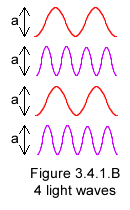Light intensity depends on 3 factual circumstances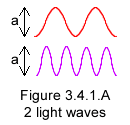Figure 3.4.1.A on the left shows 2 light waves here on Earth that are emitted by 2 electrons within our sun; 1 wave of red color, consisting of 2 wavelengths (replacing in gravimotion interpretation of Nature science's physics 2 photons) the other of violet color consisting of 4 wavelengths (replacing 4 photons).
Both waves have the same amplitude "a" peak to trough; it reflects the fact that these waves are each emitted by 1 electron, and that they travelled the same distance from Sun to Earth.
Figure 3.4.1.B on the right shows 4 waves emitted by 4 electrons within our Sun and having all the same amplitude "a" here on Earth. Because they were emitted by 4 electrons instead of 2 there are twice as many waves on the right as there are on the left, and the light, as a whole, represented on the right is twice as bright.

Light brightness or light intensity depends on 3 physical factors:1. The light intensity of each individual wave depends on the electric charge of the electron. While each wave shown above has an amplitude "a" here on Earth, it had an amplitude "Amax" greater than "a" when leaving our Sun.
2. Light intensity depends on the distance there is from the source to where the light is observed or measured.
3. Then the overall intensity of the light observed is controlled by the number of electrons emitting that light in the Sun and that coincides to as many individual waves here on Earth.
Light intensity diminishes with distance from the source (figure 3-4-1-C), in accordance to the inverse square law, and in gravimotion each individual wave obeys that law.
By contrast individual waves do not appear in science interpretation of intensity. In science light brightness is interpreted differently.
In science photons or energy quanta, which are mathematically defined as E = hν, rather than individual waves are considered. And a multitude of light quanta, rather than a multitude of individual waves, makes the intensity of light.
In that formula E is the energy of the photon, ν (Greek letter for n, pronounce "new") is the frequency of the photon, which is the very frequency of the wave it is associated to; h is Planck's constant. The frequency is treated in details further down in this page.
1. The density of the photons that is the number of photons traversing equal areas perpendicular to the direction of travel, diminishes with distance from the source; and that decrease in density obeys the inverse square law just as the wave does.
2. Unlike the wave though, the photon doesn't fade with distance; in the case chosen here, a photon on Earth has the very same energy as when it left the sun; if undisturbed while travelling, a photon keeps its energy forever. And that is expressed in the equation E = hν, in which there is no mention of distance from the source.
Note that in gravimotion's interpretation (figure 3-4-1-C) the frequencies of the waves also remain intact through distance.
In other words the sole mathematical characteristic of the photon, the frequency, is integral part of the physical wave.
The difference between science and gravimotion representations is now obvious.
The particle photon, which has no dimension and no physical body, is a mathematical entity of type energy called frequency energy.
Because the photons keep their energy forever, the decrease of light intensity through distance in science physics occurs through the scattering of photons particles in surfaces perpendicular to the direction in which the light travels
.
In gravimotion, by contrast each individual wave fades away from the source, along the distance of travel; at play is the spreading of the waves rather than a scattering of particles; it is represented with decreases of the individual waves' amplitude (figure 3-4-1-C).
In gravimotion, light waves occupy the entire volume of space; in science, photons occupy negligible (if any) volumes of space.

That highlights science's inconsistency. On one hand in science the dynamic electromagnetic field made of both wave amplitude and wave frequency does fade with distance (Maxwell equations). On the other hand the energy equivalent of that field, even though it is also made of the very same wave frequency (the photon), does not fade with distance. The frequency dubbed energy in the photon remains unchanged over distance even when the very same frequency of the very same wave (in duality) no longer exists.

In the eyes of this writer that difference justifies the dismissal of the photon entity.
Note that the ambiguity could be lifted experimentally.
That will happen the day we will be able to measure weather a single photon fades with distance as a physical wave does or if the photon keeps running unabated forever as its mathematical definition suggests.
The experiment could consist in showing that the emission of very remote violet light (violet photons in frequency) doesn't trigger any photoelectric effect; not because it travels along a path that misses the target (physics' way), but because its path is going through the target and its intensity died out (gravimotion's way).

The color of light is coincidental to its frequency akin to a pitch
The light of the sun is made of all the colors of the rainbow, which when mixed, look like white light.
A plant green in color reflects green light only; all the other colors of the solar spectrum that shine on it are filtered or absorbed or transformed by the plant.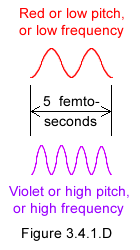The color of light is coincidental to the light wave frequency, which is the count of cycles or peaks of the wave per unit time. The color of light is equivalent to the pitch of sound. Both color and pitch coincide to frequencies, and all 3 terms: color, pitch and frequency will be used indiscriminately for light waves in the following.
Figure 3.4.1.D (on the left), just as the previous figures, represents only 2 of these colors, the red and the violet.
As shown on that figure, the red light wave has 2 cycles (2 peaks) in 5 femtoseconds, a femtosecond being a thousandth of a millionth millionth second!
Violet light has 4 cycles or 4 peaks in 5 femtoseconds.
Let's consider 1 cycle only; a full cycle of red light is twice as long as a violet cycle, see details figure 3.4.1.E on the right.
Considering the action in time that is no matter the amplitude of the wave, the action within a cycle of violet light will occur twice as fast as the action within a red cycle.
At high frequency the action occurs faster; wherever sensed, felt or measured, a high frequency wave is providing more energy than a low frequency wave.
The photoelectric effect as interpreted in gravimotion (§3.4.2)
In 1887 Hertz discovered that light rays, in some specific conditions, extract electrons out of a photoelectric material; a phenomenon known under the name of photoelectric effect, and described next.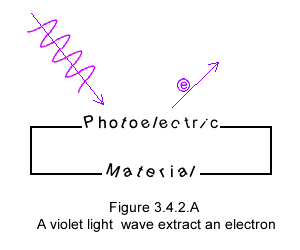A light beam of higher frequency or pitch (violet instead of red) and emitted by a single electron, when aimed at a photoelectric material, extracts 1 electron out of the material, as shown in figure 3.4.2.A; on the other hand a light beam of red frequency figure 3.4.2.B does not.
Furthermore the violet light provides a specific speed to the extracted electron.
A higher frequency than violet yet provides a faster speed to the electron. The speed of the electron is directly linked to the light frequency or pitch of the wave representing it. The higher the frequency the higher the speed of the emitted electron.
Now the intensity of the high frequency light (violet color) has a very specific effect. An increase of intensity, let say a light beam made of 2 waves as shown in figure 3.4.2.C, emits 2 electrons instead of 1, and as already mentioned all the electrons are emitted with the same specific speed, characteristic of the violet frequency.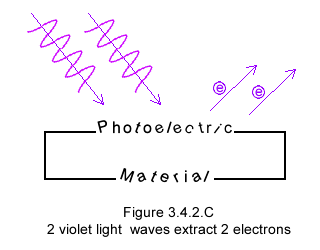On the other hand increasing the brightness of a red (or low pitch) light doesn't extract any electrons. Whether the beam of red light is made of 2 waves as on figure 3.4.2.D or a thousand waves the electrons remain stubbornly confined within the photoelectric material.
Obviously the red light pitch is too low, or too slow, to provide a sufficient speed to the electrons as to extract them out of the photoelectric material.

As one can see considering light is made of waves, the photoelectric effect is elegantly explained. Nothing in this description mandates that light be also made of particles.
Clearly the simplicity of the physical phenomenon doesn't show any conflict of interest that would justify the invention of the photon.
By contrast science's mathematical description is much more complex to the point of being incomprehensible, as it requires both interpretations of waves (Maxwell equations) and particles (Einstein's energy quantum or photon).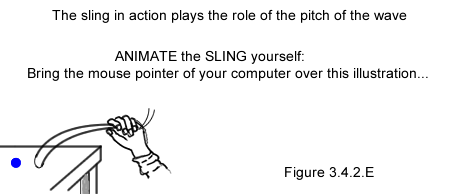The laws of physics are clear they simply confirm the photoelectric phenomenon!
A free electron within the photoelectric material, is an electron free to roam around the atoms of the material; should an electromagnetic wave catch one of these electrons that electron is sent into a circular or spiraling motion by the pitch (variation in time figure 3.4.1.E) of the wave. That spiraling motion will at most be one half a full revolution, before the electron gets to speed and gets ejected as with a sling and as shown figure 3.4.2.E. The accelerated motion of an electron cannot last longer than half a revolution as the action occurs during an increase or a decrease of the wave represented figure 3.4.1.E and which variation coincides to a variation of motion (acceleration).
Any electron will be extracted provided its motion (its energy or its speed in physics) is sufficiently high as to overcome the surface barrier of the photoelectric material. Just as a bearing-satellite-rocket speed must exceed a certain threshold as to escape earth's gravity; evidently in case of the electron the constricting energy is electrical in nature rather than gravitational.

Hence the wave constitution of light explains the photoelectric phenomenon in its entirety.

In science light remains nevertheless wave and particle at same time.

Even though, as shown above, particles light (photons) are not needed in the real world, they are needed in the mathematical world.
While no one denies the benefits of a mathematical photon, one should also recognize that the photon has no physical collateral.
Physics duality of light is as preposterous in the gravimotion world as it is irrational in reality (§3.4.3)
In science:
1. The particle photon has no dimension.
2. The particle photon has no mass.
3. The particle photon has no electric charge.
4. To make it worse, the particle photon is emitted instantaneously that is in no time.
The photon's only characteristic is energy, which ends up being a new and mysterious type of energy labeled "frequency".
The specificity of the photon is that it is not an electromagnetic wave, because if the photon was an electromagnetic wave there would be no duality.
As such the photon "frequency/energy" is not of electromagnetic type.
And because the photon has no mass that "frequency" energy cannot be associated to the force of gravity either.
Then one wonders to which of the 2 other fundamental forces is associated that type of energy that is frequency and that coincides to the point particle photon.
That same question can be rephrased another way:
Why is the photon energy not matching any of the 4 forces that are in force in science's standard model?

I have always been uneasy about mathematics; the mathematical ambiguity domineering light duality comforts that attitude of mine.
And that mathematical duality of light is not the only one bothering me. Physics' worse mathematical aberration is Michelson Morley proof that space is empty, as we know today that space called now "quantum vacuum" is instead full of frantic energy!
Yet both mathematical versions of space that is empty and full of energy at same time, are still honored in science!

On other grounds, physicists relish mentioning that a theory is justified when it includes the previous theory. Because at slow speeds it includes Newton�s theory, the relativity theory, many claim, is no doubt true.
Should we conclude that Einstein�s invention of the photon and along with it the mathematical duality of light, which now doesn't include the previous theory, which was written by Maxwell, is not true?
The precept relished by physicists, which is that the old theory be integrated in the new theory, makes utterly sense.
The photon and duality of light are denied in gravimotion though, not in compliance of above physics precept (nevertheless disregarded by physicists themselves in that specific case!) but because the above proposed physical oneness of light makes much more sense than its mathematical duality.

Even though it matches specific physical measurements, physics mathematical wave / photon duality departs greatly from Nature's elegant simplicity.
And as per Leonardo da Vinci "Simplicity is the ultimate sophistication". Let it be known that that simplicity, elegance and sophistication, all in one, concerns Nature, and not gravimotion, which is only a mental attempt at penetrating Nature.

No one denies that from the point of view of applications and predictions there is nothing like physics mathematics...
Inspired by physics science, and maybe more specifically by physics science own internal discordances, gravimotion is an interpretation of Nature, which goal is to unveil the real nature of Nature.
Should you (on your side) be thinking that my reasoning is "philosophical", you might be right...
Gravimotion is not a mathematical theory.
But then I (on my side) am thinking that physics light duality, while dressed up in well-tuned mathematics, doesn't reflect the elegance of Nature that, it seems to all of us, resides in unity rather than in various dualities.